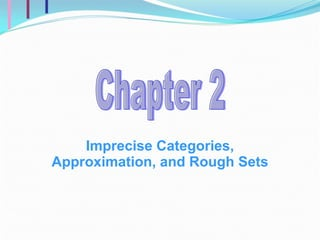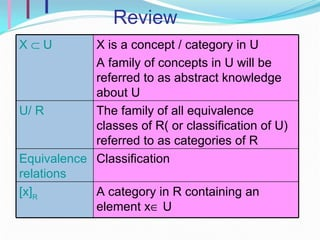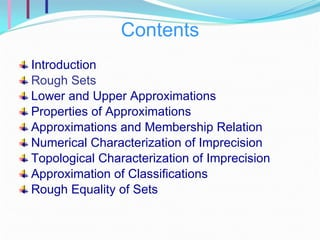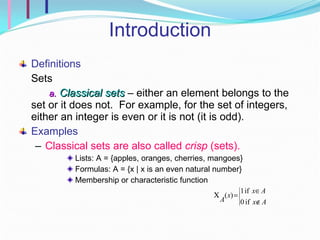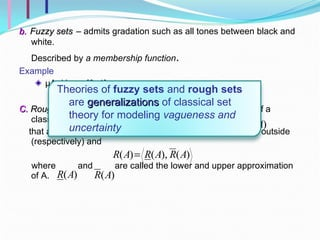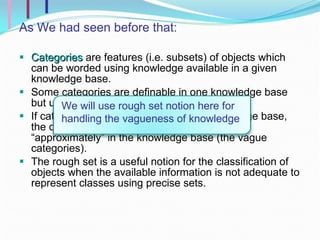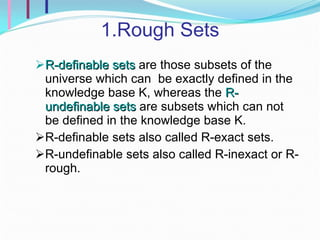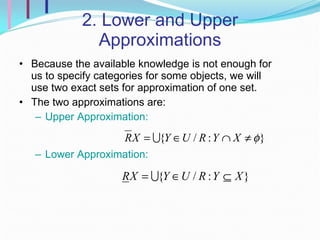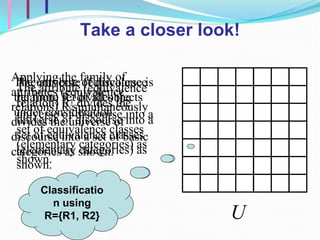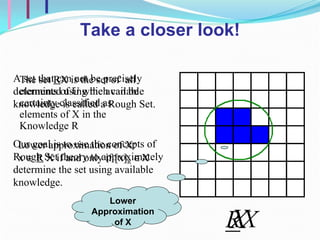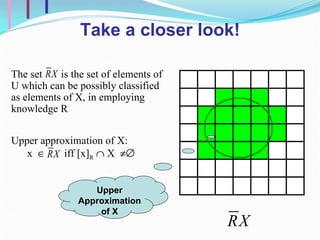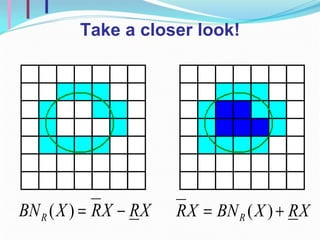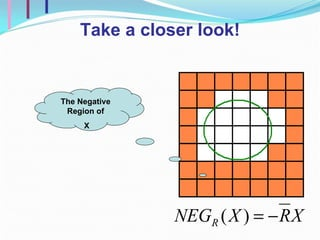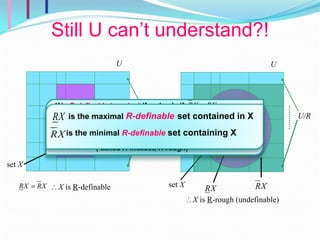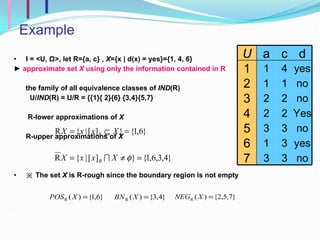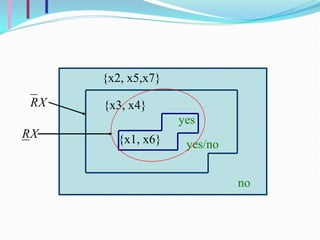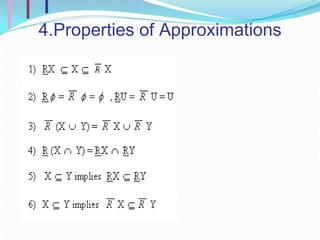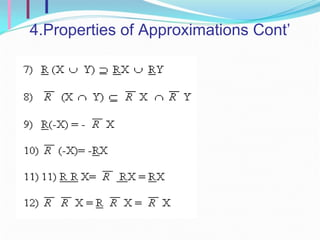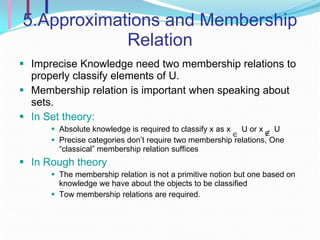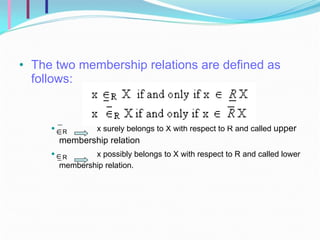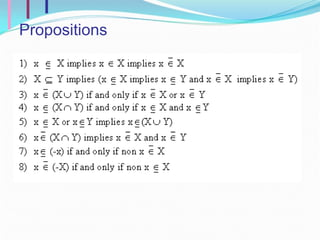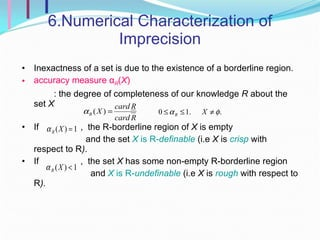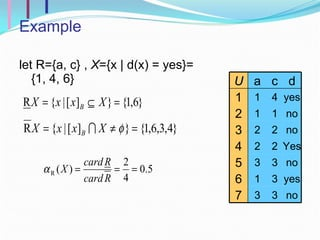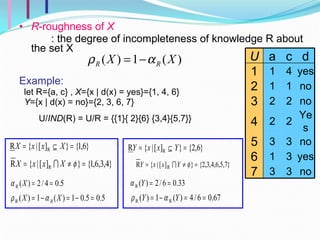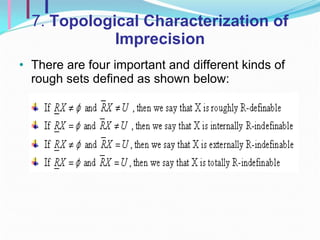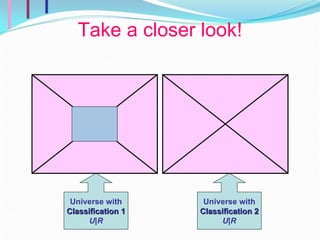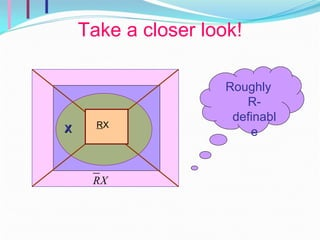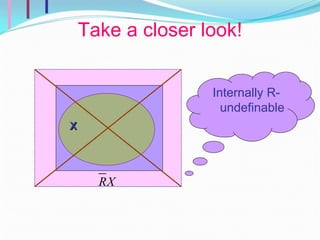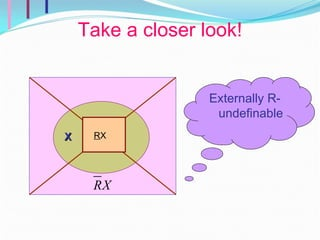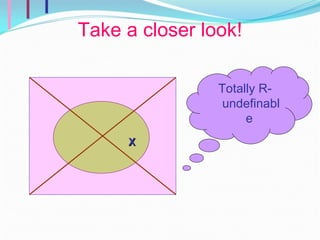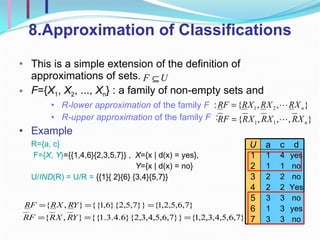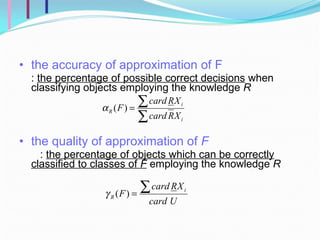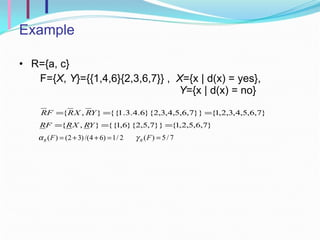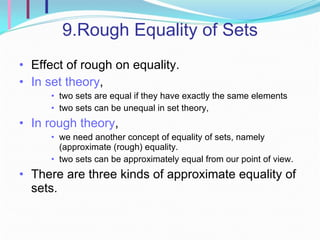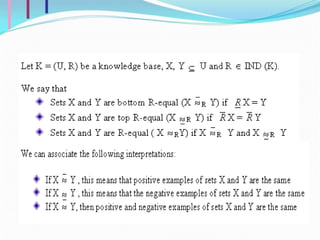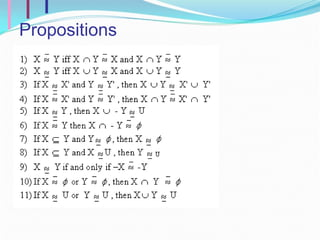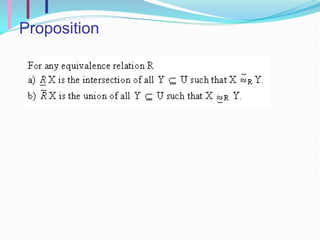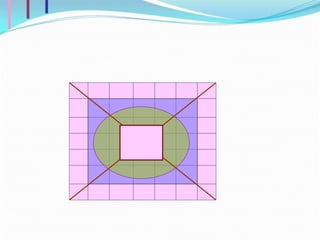1 de 38

### Chapter 2

1. Imprecise Categories, Approximation, and Rough Sets Chapter 2
2. Review Classification Equivalence relations A category in R containing an element x  U [x] R The family of all equivalence classes of R( or classification of U) referred to as categories of R U/ R X is a concept / category in U A family of concepts in U will be referred to as abstract knowledge about U X  U
3. Take a closer look! The universe of discourse is the finite set of all objects under consideration. The attribute (equivalence relation) R 1 divides the universe of discourse into a set of equivalence classes (elementary categories) as shown. Classification using R1 Classification using R2 The attribute (equivalence relation) R 2 divides the universe of discourse into a set of equivalence classes (elementary categories) as shown. Applying the family of attributes (equivalence relations) R simultaneously divides the universe of discourse into a set of basic categories as shown. Classification using R={R1, R2}
4. Take a closer look! A set that can not be precisely determined using the available knowledge is called a Rough Set. Our goal is to use the concepts of Rough Set theory to approximately determine the set using available knowledge. The set R X is the set of all elements of U which can be certainty classified as elements of X in the Knowledge R Lower approximation of X: x  R X if and only if [x] R  X Lower Approximation of X
5. Take a closer look!
6. Take a closer look! The Negative Region of X
7. Still U can’t understand?! ∴ X is R -rough (undefinable) U U/R R : subset of attributes set X ∴ X is R -definable U/R U set X X is R-definable (or crisp) if and only if ( i.e X is the union of some R -basic categories, called R-definable set , R-exact set ) X is R-undefinable ( rough ) with respect to R if and only if ( called R - inexact , R - rough ) is the maximal R-definable set contained in X is the minimal R-definable set containing X
8. yes yes/no no {x1, x6} {x3, x4 } {x2, x5,x7}
9. 4.Properties of Approximations
10. 4.Properties of Approximations Cont’
11. Propositions
12. Take a closer look! Universe with Classification 1 U|R Universe with Classification 2 U|R
13. Take a closer look! R X X Roughly R-definable
14. Take a closer look! X Internally R-undefinable
15. Take a closer look! R X X Externally R-undefinable
16. Take a closer look! X Totally R-undefinable
17.
18. Propositions
19. Proposition
20.